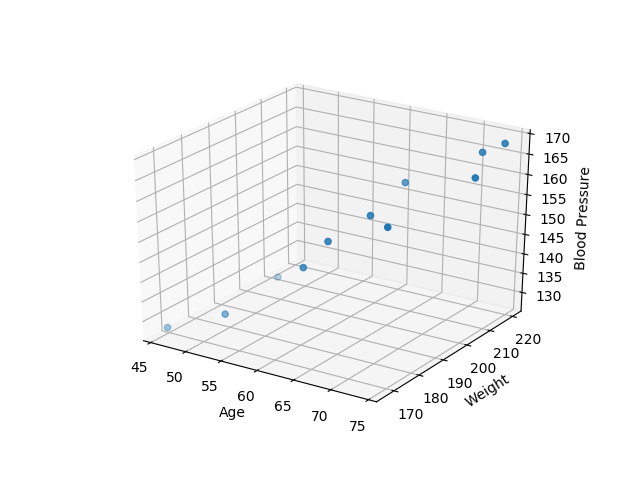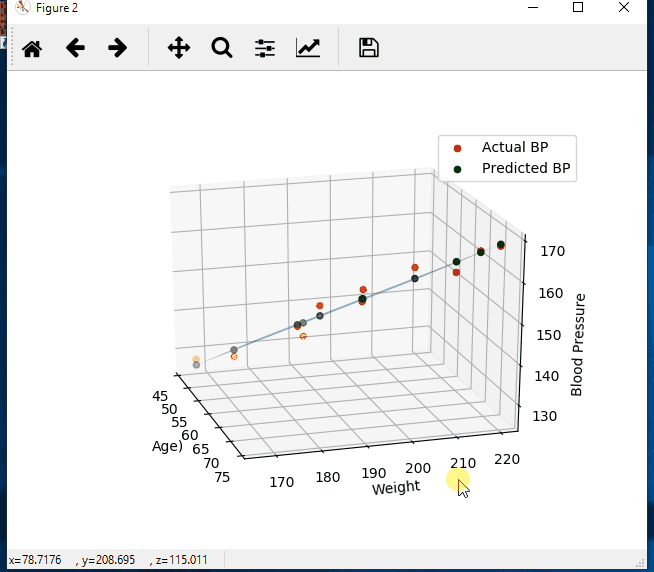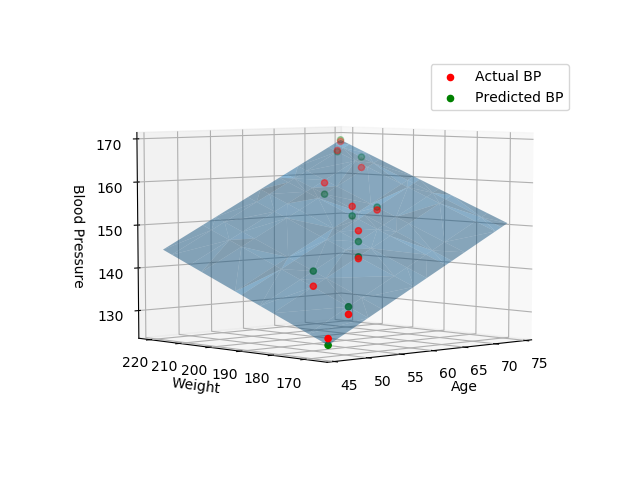# Multiple linear regression with Python, numpy, matplotlib, plot in 3d

Home Forums Linear Regression Multiple linear regression with Python, numpy, matplotlib, plot in 3d

Viewing 1 post (of 1 total)
• Author
Posts
• #1499

Background info / Notes:

Equation:
Multiple regression: Y = b0 + b1*X1 + b2*X2 + … +bnXn
compare to Simple regression: Y = b0 + b1*X

In English:
Y is the predicted value of the dependent variable
X1 through Xn are n distinct independent variables
b0 is the value of Y when all of the independent variables (X1 through Xn) are equal to zero
b1 through bn are the slope of the relationship between the dependent variable and the independed variable that is holding constant of all other independent variables.

Think of it as a system of equations:
Y1 = (b + mX1) + e1
Y2 = (b + mX2) + e2

Yn = (b + mXn) + en
We can then set up a matrix equation with the following matrices:

``````
|Y1|
Y =  |...|
|Yn|

|1 X1|
X =  |...|
|1 Xn|

|b|
A = |m|

|e1|
E = |...|
|en|
``````

Which gives us the matrix equation: Y = XA + E
We just need to solve for A

Use Linear Algebra to solve
Equation:
A = (X^T * X)^-1 * (X^T * Y)

Convert the equation to code:
Using the np.linalg.solve function we will not need to invert the first term
a = np.linalg.solve(np.dot(X.T, X), np.dot(X.T, Y))

The full code:

``````import numpy as np
import matplotlib.pyplot as plt
from mpl_toolkits.mplot3d import Axes3D

# create arrays for the data points
X = []
Y = []

y, x1, x2 = line.split(',')
X.append([float(x1), float(x2), 1]) # add the bias term at the end
Y.append(float(y))

# use numpy arrays so that we can use linear algebra later
X = np.array(X)
Y = np.array(Y)

# graph the data
fig = plt.figure(1)
ax.scatter(X[:, 0], X[:, 1], Y)
ax.set_xlabel('Age')
ax.set_ylabel('Weight')
ax.set_zlabel('Blood Pressure')

# Use Linear Algebra to solve
a = np.linalg.solve(np.dot(X.T, X), np.dot(X.T, Y))
predictedY = np.dot(X, a)

# calculate the r-squared
SSres = Y - predictedY
SStot = Y - Y.mean()
rSquared = 1 - (SSres.dot(SSres) / SStot.dot(SStot))
print("the r-squared is: ", rSquared)
print("the coefficient (value of a) for age, weight, constant is: ", a)

# create a wiremesh for the plane that the predicted values will lie
xx, yy, zz = np.meshgrid(X[:, 0], X[:, 1], X[:, 2])
combinedArrays = np.vstack((xx.flatten(), yy.flatten(), zz.flatten())).T
Z = combinedArrays.dot(a)

# graph the original data, predicted data, and wiremesh plane
fig = plt.figure(2)
ax.scatter(X[:, 0], X[:, 1], Y, color='r', label='Actual BP')
ax.scatter(X[:, 0], X[:, 1], predictedY, color='g', label='Predicted BP')
ax.plot_trisurf(combinedArrays[:, 0], combinedArrays[:, 1], Z, alpha=0.5)
ax.set_xlabel('Age')
ax.set_ylabel('Weight')
ax.set_zlabel('Blood Pressure')
ax.legend()
plt.show()``````Viewing 1 post (of 1 total)
• You must be logged in to reply to this topic.